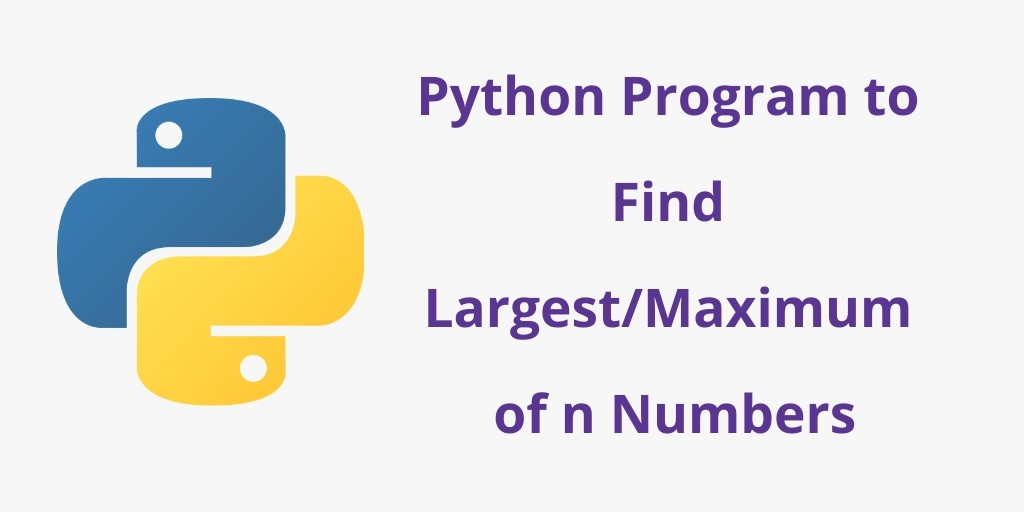# Python : Program to find maximum number – Exploring max()Hello Friends,

Today in this article we are going to discuss that how to find maximum number in Python using max() function

What is max() function?

Python max() function returns the largest item in an iterable or the largest of two or more arguments

Syntax:

``1, num2, *num [, key]) ``

Example

``````num1 = 4
num2 = 8
num3 = 2

max_num = max(num1, num2, num3)
print(max_num)
----------------------------------------
In runfile(...)
8``````

What is append() function in Python?

append() function in python that adds a single item to the existing list.

Syntax:

``````list.append(item)
``````

Example

``````currencies = ['Dollar', 'Euro', 'Pound']
currencies.append('Yen')
print(currencies)
-----------------------------------------------
In runfile(...)
['Dollar', 'Euro', 'Pound', 'Yen']

``````

Program to determine maximum number from the given numbers

Creating a empty list

``numbers = []``

Asking number of elements from which we need to find the maximum number and add to list

``count = int(input("Among how many number do you want to find")``

``````for icounter in range(1, count + 1):
digits = int(input("Enter digits: "))``````

append() function is method in python that adds a single item to the existing list.

``numbers.append(digits)``

print maximum number using max() function

``print("Largest digit is:", max(numbers))``

Full code

``````# Program to determine maximum number from the given numbers

# Creating a empty list
numbers = []

# asking number of elements to put in list
count = int(input("Amoung how many number do you want to find"))

for icounter in range(1, count + 1):
digits = int(input("Enter digits: "))

# append() function is method in python that adds a single item to the existing #list.
numbers.append(digits)

# print maximum number using max() function
print("Largest digit is:", max(numbers))
-----------------------------------------------------------------------------------
In runfile(...)

Amoung how many number do you want to find: 3
Enter digits: 7
Enter digits: 9
Enter digits: 3
Largest digit is: 9``````